# Part 1 – Direct Current

In the first part of our look at measuring voltage and current with microcontrollers, we will see how to measure DC voltage and current using an Arduino.

In part two we will do the same thing with AC, but today our focus is on Direct Current.

## Introduction

There are many reasons why you might want to use a microcontroller to measure DC voltage and current. You might be monitoring the output of a generator or solar array, you could be measuring the current consumption of your project or you might want to observe the charging and discharging of a battery.

In this article, I will show you how to measure DC voltage and current using an Arduino. The techniques here apply to any microcontroller, in fact devices like the ESP-32 or Seeeduino XIAO can actually perform better DC voltage measurements as they have analog to digital converters with a higher resolution.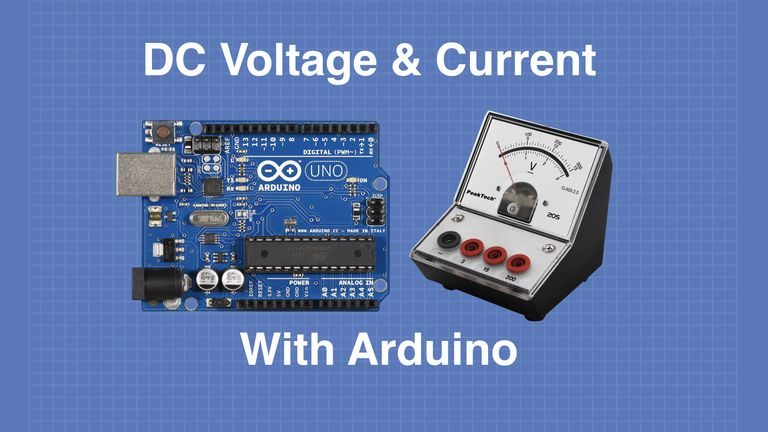Let’s get started!

## Measuring DC Voltage

Measuring DC Voltage with a microcontroller (or any digital data device) requires the use of an Analog to Digital Converter (ADC). Many modern microcontrollers, including the Arduino Uno, have a built-in ADC, making DC voltage measurement the simplest of our four tasks.

### Analog to Digital Converters

An Analog to Digital Converter is pretty well exactly what it sounds like. It’s a component that accepts an analog input and produces a digital output, the output being a digital representation of the level of the input.The accuracy of this conversion is determined by a few different factors:

Number of Bits – This determines how many “steps” the converter can divide the input into. The more boots, the more granular the output can be.Voltage Reference – The converter is only as precise as the reference voltage applied to it. This also determines the maximum voltage the ADC can accept at its input.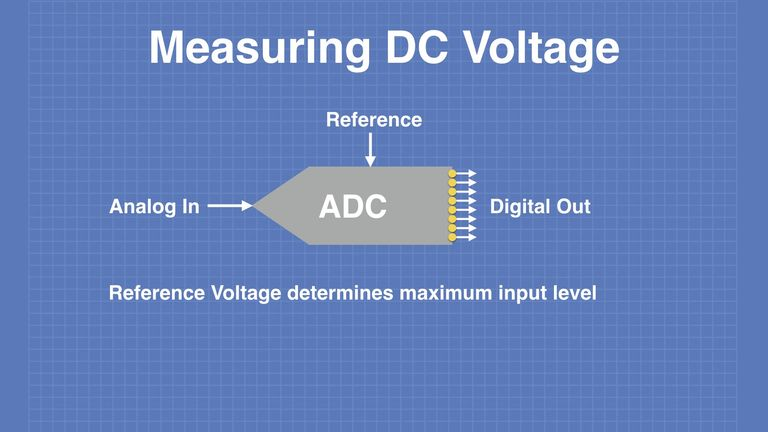Linearity – The converter needs to have a linear output, that is to say, it needs to have its output readings increase by the same amount for each increment.

Many microcontrollers, like the Arduino Uno, have built-in ADCs. The Uno has six 10-bit ADCs, meaning that they can resolve the input down to 1024 discrete steps (2 to the power of 10 equals 1024).

Other microcontrollers also have built-in ADCs, some of them with a greater resolution than the Arduino Uno. With the ESP32 and Seeeduino XIAO, we have a selection of 12-bit ADCs, allowing them to resolve the input voltage down to 4096 steps.### Voltage Dividers

The maximum input voltage that you can feed into an Arduino Uno ADC is 5-volts, with microcontrollers using 3.3-volt logic it is even less. Obviously, this is a bit impractical, as you’ll probably want to measure input voltages exceeding that.To accomplish this we can use a voltage divider, a very simple circuit constructed using two resistors. This reduces the voltage and has the added benefit of increasing the input impedance, which means that the measurement device won’t load down the circuit it is trying to measure and distort the reading.You can certainly construct a voltage divider using discrete resistors, in fact, if you are planning to measure very high voltages then you’ll probably have to. However, If you are planning on using your Arduino to measure lower voltages, less than 26-volts, you can purchase a premade divide that will do the job. These devices are extremely inexpensive and use precision resistors to give an accurate reading.

I’ll be using one of these dividers in our experiments. It consists of a 7.5k resistor and a 30k resistor.

### Voltage References

As stated earlier one of the factors that determine the accuracy of our ADC is its reference voltage.

By default, the Arduino Uno uses its power supply voltage as the reference. This is supposed to be 5-volts and can be derived either from its USB port or from its internal linear voltage regulator, which is used when the Uno is powered using the barrel connector.

These voltages are not always precise, the USB voltage is dependent upon your computer’s power supply and the length and gauge of the USB cable itself. When using the linear regulator with an external supply the accuracy is determined by that regulator,, which can also vary from exactly 5-volts.

As long as the voltage is between 4.75 and 5.25 volts the Arduino logic circuitry will be happy. But that variance can affect the accuracy of the analog to digital converter when it’s used as a reference.The Arduino Uno is also capable of using an external voltage reference, which is connected to the AREF pin. This reference voltage cannot exceed 5-volts.

In order to use the external reference in your code, you need to specify it using the analogReference command. This command lets the Arduino know that it should use the external reference on the AREF pin instead of the supply voltage.

You’ll note from the link above that there are actually multiple parameters you can give to analogReference, however not all microcontrollers support them:

• DEFAULT – Use the microcontroller’s power supply voltage as a reference. If you don’t specify anything then this is what will be used.
• EXTERNAL – Use the external voltage reference applied to the AREF pin.
• INTERNAL – Some microcontrollers also have an internal precision reference. The link above explains this in detail.

By using the correct external reference voltage you can improve the performance of the Arduino ADC.

## Measuring DC Voltage with the Arduino

We will begin our experiments by measuring DC voltage. And the Arduino Uno has six 10-bit ADCs we could actually measure multiple voltages simultaneously, but today we’ll just focus on using one input and measuring a single voltage.

### Arduino Hookup 1 – Basic DC Voltage Measurement

The hookup for our first experiment is very simple:All we are doing here is using a resistive voltage divider in front of one of the analog inputs on the Arduino. Here is an image of that divider, which is commonly available at just about any electronics store: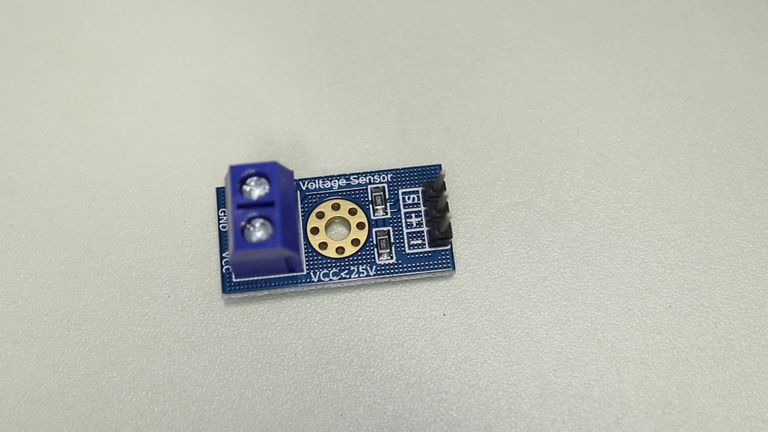If you don’t have one of these you can use a couple of resistors. The divider I used has two resistors:

• A 30k resistor on the “high-side”, the connection between the output and the input voltage.
• A 7.5k resistor between the output and ground.

This will reduce the voltage input by about a factor of 5.

You can also calculate your own divider if you want to measure higher voltages.

### Arduino Sketch 1 – Basic DC Voltage Measurement

Here is the sketch we are using for our DC voltage measurement experiment:

This is a pretty simple sketch, so you should have no problem understanding it.

After defining the analog input pin we are using we declare a few floats that represent the voltage at the input of the ADC, as well as the voltage we are trying to measure on the input of the divider.

We then declare the values of the resistors in the divider. If you are making your own divider with different resistors you’ll need to change this.

We define a float for the reference voltage, which in this case is 5-volts as we are using the Arduino’s power supply voltage as a reference.

And the last variable we define is the value produced by the analog to digital converter, which is an integer that can range from 0 to 1023.

We will display our results on the serial monitor, so we define that in the Setup.

In the loop, we read the value from the ADC and then calculate the voltage level based upon that. We then factor in the resistors to determine the actuarial input voltage to the divider.

After that we just print the results to the serial monitor, add a delay and then do it all again.If you have a multimeter (and you should have one if you work with this stuff) measure the voltage and compare the measurement to what you see on the serial monitor. Depending upon how close to 5-volts your Arduino power source is you may or may not see the same value.

Let’s see if we can improve that!

### LM4040 Precision Voltage Reference

In order to improve the performance of our basic DC voltage measurements, we will need to provide our Arduino with an external voltage reference, which we will connect to the AREF pin.

There are many different reference chips and modules you can choose from. I have decided to use one from Adafruit that I think is pretty cool.The LM4040 is a precision regulator chip, and this module actually contains two of them. They are arranged to provide two precision outputs:

• 4.096 VDC – Ideal for 5-volt microcontrollers like our Arduino Uno.
• 2.048 VDC – Great for 3.3-volt devices like the ESP32 or Seeeduino XIAO.

At first glance you might find those to be oddball values until you look at them in millivolts – they are 4096 and 2048 mv respectively. Considering your ADC has resolutions of 1024 or 4096 parts it suddenly makes perfect sense!

So our Arduino Uno ADC with a 10-bit (1024 parts) resolution would be measuring 4mv for each increment.

Let’s use this device with our circuit and see if it helps with the accuracy.

### Arduino Hookup 2 – DC Voltage Measurement with LM4040

Here is the revised hookup for our DC voltage measurement experiment:Note that the only change is the addition of the LM34040 module, which is powered from the 5-volt output of our Arduino

### Arduino Sketch 2 – DC Voltage Measurement with LM4040

Here is the sketch we will be using to measure DC voltage with an external voltage reference.

Note that this sketch is virtually identical to the previous one, in fact, it only has two differences:

• The reference voltage has been set to 4.096.
• The analogReference command specifies an EXTERNAL voltage reference.

So load this sketch to the Arduino and give it a test.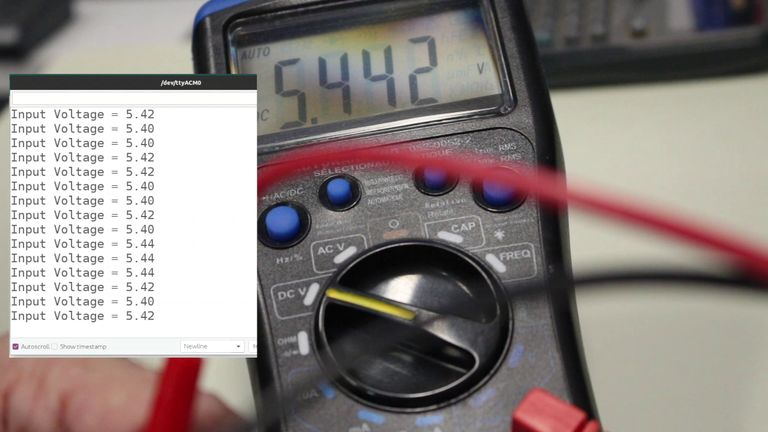I noticed a great improvement over the previous sketch, which was to be expected.

BTW, you can also use the LM4040 to test the accuracy of your multimeter. I was actually surprised at how accurate my inexpensive DMM was!

## Measuring DC Current

Now that we have seen how to measure voltage with our Arduino let’s turn our attention to the measurement of current.

Measuring current usually involves turning that current into a voltage, which can then be measured using an ADC as we did above. There are a couple of different ways of accomplishing this.

### Invasive vs. Non-Invasive Techniques

We can divide our current measurement techniques into two categories – Invasive and Non-invasive.

An invasive method is inserted in series with the circuit whose current we wish to measure. It is “invasive” as its insertion slightly affects the performance of the circuit itself, although this effect is usually fairly trivial.

A non-invasive method does not require any direct connection to the circuit. Instead, the magnetic field that the current produces in a conductor is measured. If you have seen (or own) those clamp-on meters this is how they operate.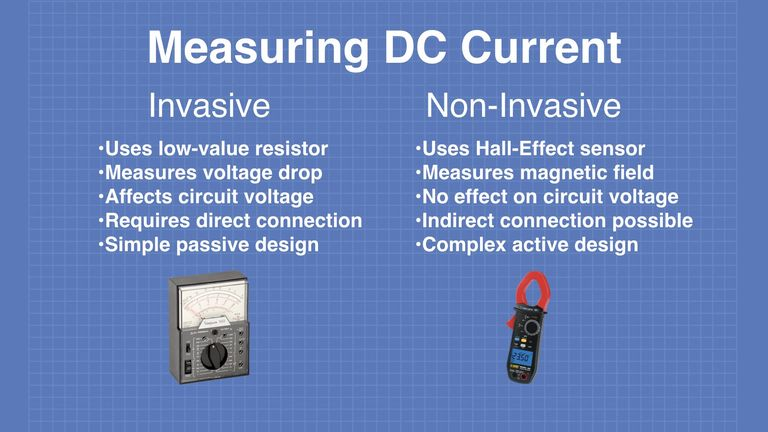Most invasive methods use a low-value resistor, which is wired in series with the circuit whose current you wish to measure. The voltage drop across the resistor is measured and Ohm’s Law is used to calculate the current flow.

The most common noninvasive method for measuring DC current involves the use of a Hall Effect sensor. This is placed near a conductor to measure the magnetic field surrounding the conductor. That field strength is used to determine the current.

Although you can obtain Hall-Effect sensors that don’t require a connection to the circuit, the one we will be using does, as the conductor whose field strength it measures is internal to the IC chip on the sensor.

## Measuring DC Current with the Arduino

We will use both methods, invasive and noninvasive, to measure current with our Arduino. We will begin with a very commonly available noninvasive sensor, the ACS712.

### ACS712 Hall Effect Sensor

The ACS712 has been around for years, in fact, it has been superseded by other models and is no longer used for new designs. However it is still commonly available and, best of all , is extremely inexpensive.

This hall-effect current sensor can be used with both DC and AC current, although today we will focus on its use with Direct Current.

This device operates on a 5-volt power supply and outputs a voltage proportional to the current it is measuring. The input connection is isolated from the output, making it safe to use for high voltage applications.

There are actually three versions of this sensor, they differ in the maximum current they can measure:

• 5 Amp
• 20 Amp
• 30 Amp

As most modules don’t have marking identifying which version they are using you’ll probably need to look directly at the ACS712 chip itself to determine this.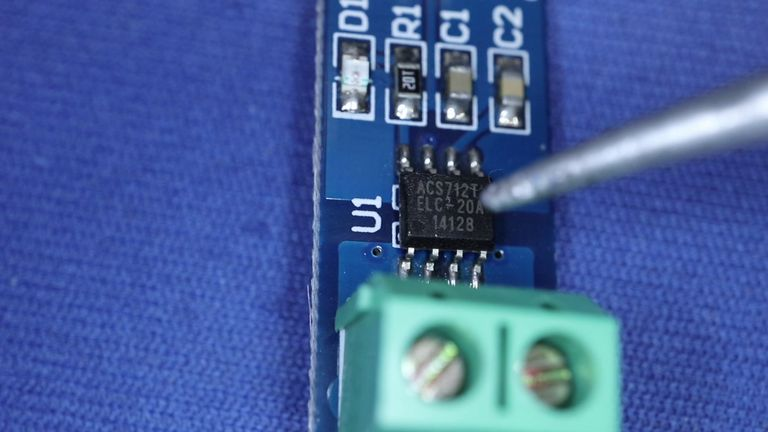In the above example, you’ll see that this is a 20-ampere version.

The ACS712 outputs a voltage of 2.5-volts when NO current is detected. Voltage above this is a positive flowing current, while below 2.5-volts indicated a negative current.

Because there are three versions of this chip you need to use a “scale factor” to determine the current, as shown here: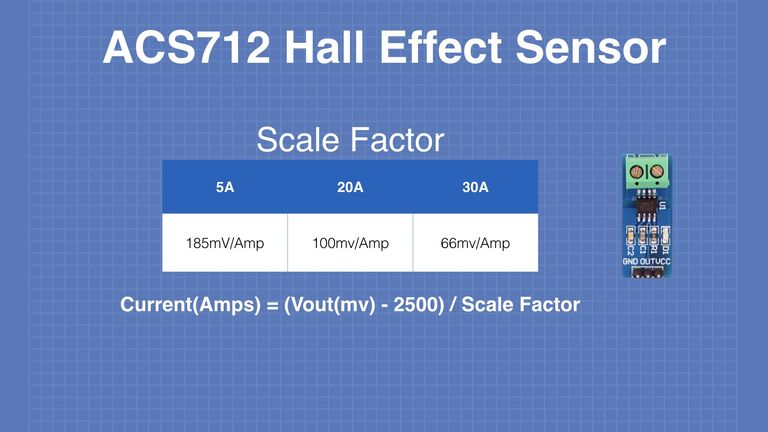The formula food determining the current is also shown in the illustration.

### ACS712 Hall Effect Sensor Hookup

Here is how we will be hooking up the ACS712 to our Arduino Uno:It’s a pretty simple hookup, the ACS712 output is connected to the Arduino analog input.

Note that the input connections to the ACS712 are not marked with polarity, as it can read both positive and negative current. If you hook it up as per the above diagram your readings will be positive.

### ACS712 Hall Effect Sensor Sketch

Here is the sketch we will be using to measure DC current with the ACS712 hall-effect current sensor:

Most of the sketch involves reading the analog input voltage and using math to determine the current.

The resADC variable is calculated using the voltage reference, which with our hookup is 5-volts. If you want to build a more accurate sensor you can modify it to use the LM4040 module we used earlier, if so you’ll want to change the value of vRef accordingly.

Also note that the zeroPoint is determined by the power supply voltage from the Arduino, and not by the voltage reference. You can change this to 2.50 if you modify the circuit for an external reference.

You’ll want to make sure your ACS712 scale factor is accommodated, otherwise, the readings will be meaningless. I show the 20 amp version of the ACS712 being used in this sketch, but I’ve also provided the values you need for the other two versions.

In the loop we read the value a thousand times, so we can get a good average. We then use the formula you saw earlier to convert the voltage reading into current. And then we display it on the serial monitor.Load the sketch to your Arduino and observe your current readings. Mine were off slightly, but I could see that with no current flowing I was still getting a slight reading, which accounted for the error. This was likely due to two factors:

• The voltage reference was the DEFAULT, using an external reference like the LM4040 module would have helped here.
• The 5-volts supplied to the ACS712 may have also been off a bit, affecting the zero-point.

But all in all, it wasn’t off by too much, so this is a pretty easy way to measure DC current (and, as you’ll see in a future article, AC current as well).

### Adafruit INA219 Current & Voltage Sensor

The final sensor that we will be using today is quite different from the others we have seen so far.

The Adafruit INA219 sensor is capable of measuring both DC voltage and current simultaneously. It also doesn’t have an analog output like aloof the other devices we have used so far, instead, this device has an I2C output. This makes it suitable for not only microcontrollers but also for microcomputers.It is actually available in two form factors, a stand-alone module and one made for use with the Adafruit Featherwing processors. Either one would be suitable for our experiment.

One great advantage about this module is that it is inserted on the “high side” of the circuit instead of the ground side, which is how many of those Voltage and Current display modules are wired. This makes it ideal for use as a voltage and current display, it would be a great replacement for the meter I used in the Convert an ATX Computer Supply to a Bench Power Supply article I did a while back.

The device has an internal 12-bit ADC and can measure up to 3.2 amperes. It is an intrusive sensor, using a 0.1-ohm precision resistor. If you’re a true hacker you can replace the resistor with another one to change the range, personally, I’m not that brave!

The Adafruit INA219 operates on a power supply of 5-volts, which can be supplied by the Arduino.

### Adafruit INA219 Current & Voltage Sensor Hookup

Here is how we will hook up our Adafruit INA219 sensor to an Arduino: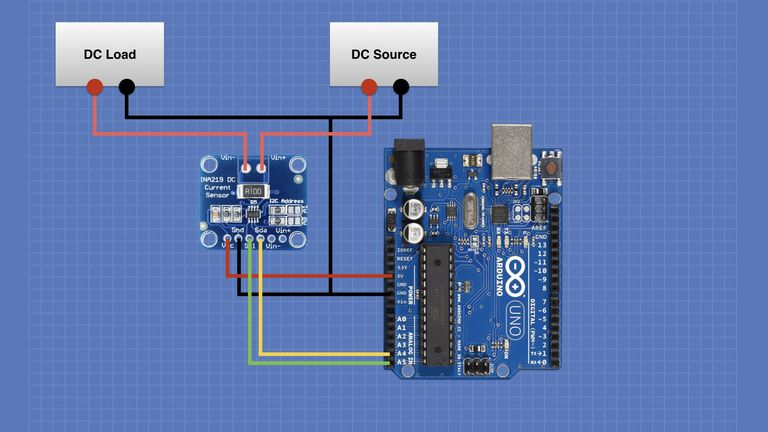Note that this is an I2C device so the A4 and A5 pins are being used as SDA and SCL, not as analog inputs.

### Adafruit INA219 Current & Voltage Sensor Sketch

In order to use this device, you’ll need to install a library that Adafruit has provided.

In your Library Manager do a search for “Adafruit INS219”. You’ll get one result, a library that you need to install. You’ll also be prompted to install some dependent libraries if you haven’t installed them previously, you’ll want to go ahead and do that.

Once you have the library installed you can run the example sketch provided by Adafruit. Go into the Examples From Custom Libraries and look for the Adafruit INA219.

Open the “getcurrent” sketch, which is shown here:

Thanks to the library, using this sensor is very simple. In the sketch, an object called “ina219” is defined, and its properties have everything you need, no calculations required. Of course, the sketch also uses the Arduino Wire library, as it is an I2C device.

The sketch prints out a number of parameters, including voltage, current, power, and the actual voltage measured across the resistor.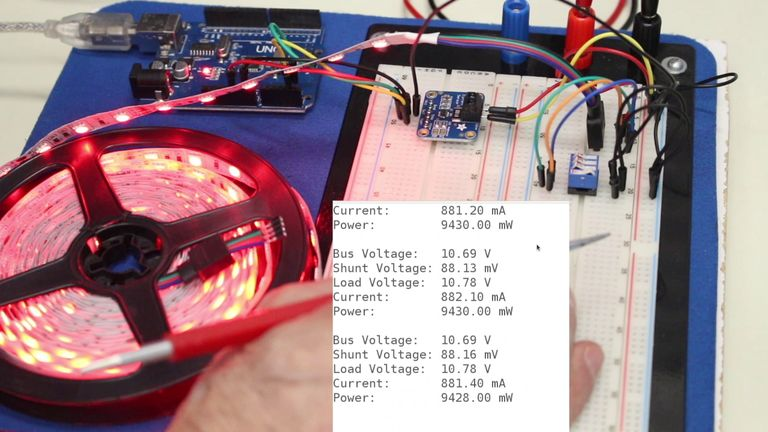Load it up and take a look, making sure that your load doesn’t exceed 3.2 amps. You should find that it works very effectively, and as it uses its own internal ADC it is not affected by the Arduino ADC voltage reference.

## Conclusion

As you have seen it is fairly simple to measure DC voltage and current using an Arduino, and you can expand these sketches to use other microcontrollers.

This is the first of a two-part series on measuring voltage and current, next time we will see what is required to do the same trick using Alternating Current.

Until then happy measuring!

### Resources

Code for this article – All of the sketches used today, in an easy to use ZIP file.

Arduino analogReference – The Arduino analogReference command allows you to use different voltage references for your Arduino ADC. This link also shows features for other processors as well.

Adafruit INA219 – A detailed article from Adafruit for using the INA219 Voltage and Current sensor.

Voltage Divider Calculator – make your own custom voltage dividers.

Measure DC Voltage and Current with an Arduino
SummaryArticle Name
Measure DC Voltage and Current with an Arduino
Description
Learn how to measure DC voltage and current using an Arduino. The first article in a two-part series, the subsequent article will deal with AC.
Author
Publisher Name
DroneBot Workshop
Publisher Logo
Tagged on:

#### If you have a question...

You are much more likely to get answers to technical questions by making a post on the DroneBot Workshop Forum. Your post will be seen not only by myself, but by a large group of tech enthusiasts who can quickly answer your question. You may also add code samples, images and videos to your forum posts.

Inline Feedbacks
Bruce
8 months ago

Great video as always. I really like the effort you also put into the attached articles.

Michael
8 months ago

Bill,

I have made your project with Arduino Nano and Adafruit INA219. The source load (Voltage) is 12.73 V. The Bus voltage shows between 0.95 V – 1.06 V.

What will be wrong in the example sketch for me?

Loïc
8 months ago

Marcin Sosna
8 months ago

Hi. If I wanted to measure different voltages (ranges: 0-5V, 0-30V etc.) on, for example, three analog inputs, can I just use other analog input voltage divider resistors and a single LM4040 module as the reference voltage?

Johnsonn
8 months ago

For INA219, can the load be the microcontroller itself if I want my mcu to go into sleep mode when the voltage/current drop to a certain level?

Glen
8 months ago

As with all your videos, one word – outstanding. That being the case, any idea when the AC version will be released?

Thanks a lot!

John doggett
6 months ago

Just a little confused, I want to run a project with a battery supply and I want to monitor the battery voltage so I know when the batteries need changing.
If I use a voltage divider, where does the power come from to power the Uno on the Vin pin.
The dividers + output is not connected so I’m confused where the uno gets power.
If I’m being thick I’m sorry for.my stupidity.

Krishna Puvvala
6 months ago

Did you manage to find the solution for your query? I’m also working on something similar but couldn’t managed to find anything useful.

John Doggett
6 months ago

Hi
There doesn’t seem to be any answers to this question, my only guess is that you run another wire from the battery positive input from the divider and connect that to the Vin pin on the Uno or nano.
It just seems a pointless module to get a voltage readout from a battery source and still use the usb to power the uno/nano.
Still scratching my head i’m afraid.
I’m going to have another sit down and test this and see if that’s the way to go’

Sabri
3 months ago

Hi. Email me exactly what you want and I can help you.

Mirol
4 months ago

Hi, can you teach me how to combine code voltage sensor and current sensor? Cause i really don’t know how to combine the code.

lupin
3 months ago

why my current output at serial monitor is negative someone help me. my email mohdnizar4@yahoo.com

Jeri
3 months ago

thanks a lot, really appreciate to dronebotworkshop

richie
3 months ago

you are just tooo great and expert…

reyaza
1 month ago

Hi Sir, if I want to montor the voltage from each cell from a 6S Lipo battery,then I would have to use all 6 ADC’s from the Arduino Uno. Is that correct ? Or is there another solution ?

16
0